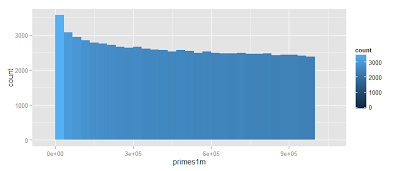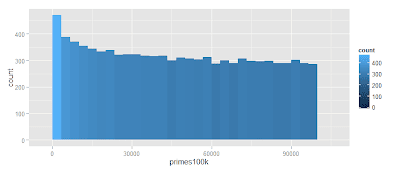## Wednesday, May 22, 2013

### My Prime Sieve - Homage to Yitan Zhang

```# As a homage to Yitang Zhang who has proven a mind-bending property of Prime Pairs, I have written a prime Sieve to detect all of the prime numbers from 1 to N.

# There might very well be a function in the base package that already does this.  No doubt there are a dozen math packages out there which does this.  However, it is the first time I have programmed a Prime Sieve :)

# A prime sieve is a simple algorithm which grabs the first number after 1 and eliminates all numbers devisible by it.  Then it grabs the next number in the set remaining and does the same for that.

primes = function(n=1000, printProgress=F) {
# 1 is always in the list
prime = 1
# The availabe set we look at as greater than 1 up to n
set = 2:n
# Loop through the set dropping anything which is not a prime and the primes as we get to them as well
while (length(set)>0) {
# Add the first number we encounter to our prime list
prime = c(prime, set)
#
set = set[floor(set/set)!=set/set]
if (printProgress) print(paste("Elements Remaining: ",length(set)))
}
return(prime)
}

# This works pretty fast.
primes1k = primes(printProgress=T)
# R finds the primes of the first 1,000 integers takes a little longer

# See it mapped out
require(ggplot2)
qplot(primes1k) + geom_histogram(aes(fill = ..count..))# To look at this idea of prime pairs lets, look at the
primes100k = primes(10^5, printProgress=T)
qplot(primes100k) + geom_histogram(aes(fill = ..count..))
# Finding the primes of the first 100,000 numbers takes much longer# There is a bit of a stretch near the beginning in which there is between 40,000 and 70,000 elements left in the remaining set in which identification of primes does not eliminate any more than a few elements from the set.  After 40,000 elements things start speeding up because the list gets shorter and is able to be scanned faster.
primes1m = primes(10^6, printProgress=T)
qplot(primes1m) + geom_histogram(aes(fill = ..count..))length(primes1m)
# This identifies 78,499 prime numbers between 1 and 1 million.

10^6/length(primes1m)
# If primes were distributed evenly they would be on average 12.7 numbers apart.

# Yitan Zhang proves the astonishing fact that there are in infinite number of primes no farther than the distance of 70 million.  This is true even when pairs of primes might be a great deal further than that from other pairs.

# There are no known uses of this theory.  However, once again a mathematician has proved something fundamental about numbers which might aid humananity in the distant future.  Currently Fermat's little theorem is widely used as the basis for modern cryptography.  Perhaps, Yitan Zhang's will be the basis for equally important work in the future.```
```
```
Syntax Highlighting by Pretty R at inside-R.org

1.Nice primes! You might like a sieve I wrote. It's a little bit faster.

sieve = function(N = 10000) {
np = rep(TRUE, N) # all odd numbers are tentatively prime

for (i in 1:sqrt(N)) {
k = i + (2*i+1) * (1:(N/(2*i+1))) # factors to cross off
k = k[ k <= N] # don't create NAs by going off the end of the vector
np[k] = FALSE # cross of the numbers with factor of 2i*+1
}

p = (2*(1:N)+1)[np] # pull out the primes by index
# p = which(np)*1+2 # alternate version
p = c(2,p) # add the even prime
nump = 1+cumsum(np) # count them
p
}

system.time(sieve(1e6) -> p) # about 0.5s for primes < 2e6 on my macbook

1.Wow that is slick!

Thanks for the comment. Far faster than mine :)

2.primes sieves are always fun!

#DETERMINE ALL PRIMES BETWEEN 1 and num
#Initialize vectors
NUMBER <- seq(2, 2000000, 1)
PRIMES <- c()

#Define
countPrimes <- function(number, primes)
{
return(list(c(primes, number), number[number %% number != 0]))
}

#Initialize
RESULT <- countPrimes(NUMBER, PRIMES)

#Run
while(min(RESULT[]) < sqrt(max(NUMBER)))
{
RESULT <- countPrimes(RESULT[], RESULT[])
}

PRIMES <- c(RESULT[], RESULT[])

This is one that I wrote. It calculates the primes between one and 2,000,000 in a couple of seconds.

3.Yitang Zhang has proven that there are infinitely many pairs of primes at most 70 million numbers apart. This is very different from saying that no two primes are more than 70 million apart – it is actually very easy to prove that there are arbitrarily long sequences of composite numbers.

1.Very good point. Sorry about the error.